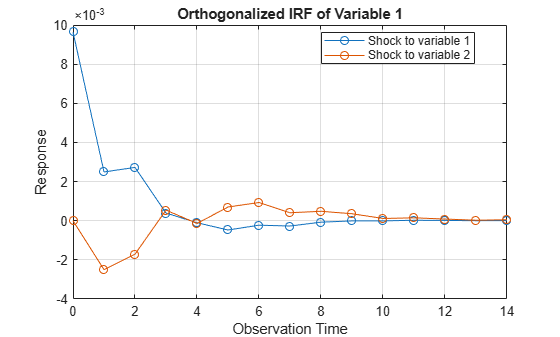# Generate VAR Model Impulse Responses

This example shows how to generate impulse responses of an interest rate shock on the US real gross domestic product (GDP) using `armairf`.

Load the `Data_USEconModel` data set. This example uses two quarterly time series: the logarithm of real GDP and the real three-month T-bill rate, both differenced to be approximately stationary. Suppose that a vector autoregression model containing the first four consecutive lags (VAR(4)) is appropriate to describe the time series.

```load Data_USEconModel DEF = log(DataTable.CPIAUCSL); GDP = log(DataTable.GDP); rGDP = diff(GDP - DEF); % Real GDP is GDP - DEF TB3 = 0.01*DataTable.TB3MS; dDEF = 4*diff(DEF); % Scaling rTB3 = TB3(2:end) - dDEF; % Real interest is deflated Y = [rGDP,rTB3];```

Fit a VAR(4) model to the data.

```Mdl = varm(2,4); Mdl.SeriesNames = {'Transformed real GDP','Transformed real 3-mo T-bill rate'}; EstMdl = estimate(Mdl,Y);```

Compute and plot the orthogonalized impulse responses in one `armairf` call by additionally returning the handle to the plotted graphics objects (the second output). Specify the estimated innovation covariance matrix.

`[YIRF,h] = armairf(EstMdl.AR,{},'InnovCov',EstMdl.Covariance);``YIRF` is a 15-by-2-by-2 array of impulse responses. Each row corresponds to a time in the forecast horizon (0,...,14), column `j` corresponds to a shock to variable Y`(:,j)` at time 0, and page `k` contains the impulse responses of a variable `Y(:,k)`.

Obtain the deflated GDP impulse response series resulting from a shock to the interest rate series by undoing the scaling of the real GDP impulse response series.

`defGDPImp = ret2price(YIRF(:,2,1));`

Plot the deflated GDP impulse response series on the original scale.

```figure; numobs = size(defGDPImp,1); plot(0:numobs - 1,defGDPImp); title('Impact of Shock to Interest Rate on Deflated GDP') ylabel('Deflated GDP'); xlabel('Time');```The graph shows that an increased interest rate is associated with a small dip in the deflated GDP series for a short time. Then the real GDP begins to climb again, reaching its former level in about three years.